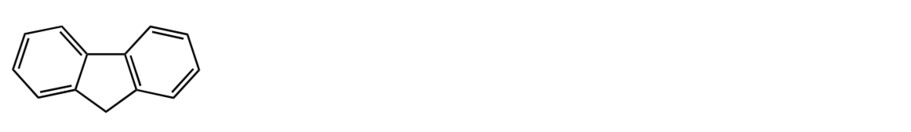# Problem: A student was searching the web for the pKa value of fluorene (see below). He found two contradicting numbers: 2 and 22. Since this is probably a typographical error, he will need to decide which value is correct. Help the student decide. Provide a  short explanation and a chemical equation to support it.

🤓 Based on our data, we think this question is relevant for Professor Gandler's class at CSU.

###### Problem Details

A student was searching the web for the pKa value of fluorene (see below). He found two contradicting numbers: 2 and 22. Since this is probably a typographical error, he will need to decide which value is correct. Help the student decide. Provide a  short explanation and a chemical equation to support it.What scientific concept do you need to know in order to solve this problem?

Our tutors have indicated that to solve this problem you will need to apply the Acidity of Aromatic Hydrocarbons concept. You can view video lessons to learn Acidity of Aromatic Hydrocarbons. Or if you need more Acidity of Aromatic Hydrocarbons practice, you can also practice Acidity of Aromatic Hydrocarbons practice problems.

What is the difficulty of this problem?

Our tutors rated the difficulty ofA student was searching the web for the pKa value of fluoren...as medium difficulty.

How long does this problem take to solve?

Our expert Organic tutor, Chris took 6 minutes and 5 seconds to solve this problem. You can follow their steps in the video explanation above.

What professor is this problem relevant for?

Based on our data, we think this problem is relevant for Professor Gandler's class at CSU.